xMoment (physics)Encyclopedia
In physics
Physics
Physics is a natural science that involves the study of matter and its motion through spacetime, along with related concepts such as energy and force. More broadly, it is the general analysis of nature, conducted in order to understand how the universe behaves.Physics is one of the oldest academic...

, the term moment can refer to many different concepts:
• Moment of force (often just moment) is the tendency of a force to twist or rotate an object; see the article torque
Torque
Torque, moment or moment of force , is the tendency of a force to rotate an object about an axis, fulcrum, or pivot. Just as a force is a push or a pull, a torque can be thought of as a twist....

for details. This is an important, basic concept in engineering and physics. A moment is valued mathematically as the product of the force and the moment arm. The moment arm is the perpendicular distance from the point of rotation, to the line of action
Line of action
In physics, the line of action of a force F expresses the geometry of how F is applied. It is the line through the point at which F is applied and along the direction in which F is applied....

of the force. The moment may be thought of as a measure of the tendency of the force to cause rotation about an imaginary axis through a point. (Note: In mechanical
Mechanical engineering
Mechanical engineering is a discipline of engineering that applies the principles of physics and materials science for analysis, design, manufacturing, and maintenance of mechanical systems. It is the branch of engineering that involves the production and usage of heat and mechanical power for the...

and civil engineering
Civil engineering
Civil engineering is a professional engineering discipline that deals with the design, construction, and maintenance of the physical and naturally built environment, including works like roads, bridges, canals, dams, and buildings...

, "moment" and "torque" have different meanings, while in physics they are synonyms. See the discussion in the "torque" article, or the article couple (mechanics)
Couple (mechanics)
In mechanics, a couple is a system of forces with a resultant moment but no resultant force. Another term for a couple is a pure moment. Its effect is to create rotation without translation, or more generally without any acceleration of the centre of mass.The resultant moment of a couple is called...

.)

• The moment of a force can be calculated about any point and not just the points in which the line of action of the force is perpendicular. Image A shows the components, the force F, and the moment arm, x when they are perpendicular to one another. When the force is not perpendicular to the point of interest, such as Point O in Images B and C, the magnitude of the Moment, M of a vector F about the point O is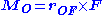where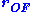is the vector from point O to the position where quantity F is applied.
× represents the cross product
Cross product
In mathematics, the cross product, vector product, or Gibbs vector product is a binary operation on two vectors in three-dimensional space. It results in a vector which is perpendicular to both of the vectors being multiplied and normal to the plane containing them...

of the vectors.

• Image C represents the vector components of the force in Image B. In order to determine the Moment, M of a vector F about the point O, when vector F is not perpendicular to point O, one must resolve the force F, into its horizontal and vertical components. The sum of the moments of the two components of F about the point O is :

M OF = F * sin (θ) * x + F * cos(θ) * 0

The moment arm to the vertical component of F is a distance x. The moment arm to the horizontal component of F does not exist. There is no rotational force about point O due to the horizontal component of F. Thus, the moment arm distance is zero, or 0.

Thus M can be referred to as "the moment M with respect to the axis that goes through the point O, or simply "the moment M about point O". If O is the origin
Origin (mathematics)
In mathematics, the origin of a Euclidean space is a special point, usually denoted by the letter O, used as a fixed point of reference for the geometry of the surrounding space. In a Cartesian coordinate system, the origin is the point where the axes of the system intersect...

, or, informally, if the axis involved is clear from context, one often omits O and says simply moment, rather than moment about O. Therefore, the moment about point O is indeed the cross product,,

since the cross product = F * x sin (θ)

• When F is the force, the moment of force is the torque as defined above.

• The Principle of moments is if an object is balanced then the sum of the clockwise moments about a pivot is equal to the sum of the anticlockwise moments about the same pivot.
• A pure moment is a special type of moment of force. See the article couple (mechanics)
Couple (mechanics)
In mechanics, a couple is a system of forces with a resultant moment but no resultant force. Another term for a couple is a pure moment. Its effect is to create rotation without translation, or more generally without any acceleration of the centre of mass.The resultant moment of a couple is called...

.
• Moment of inertia
Moment of inertia
In classical mechanics, moment of inertia, also called mass moment of inertia, rotational inertia, polar moment of inertia of mass, or the angular mass, is a measure of an object's resistance to changes to its rotation. It is the inertia of a rotating body with respect to its rotation...

(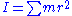) is analogous to mass
Mass
Mass can be defined as a quantitive measure of the resistance an object has to change in its velocity.In physics, mass commonly refers to any of the following three properties of matter, which have been shown experimentally to be equivalent:...

in discussions of rotational motion.
• Moment of momentum or angular momentum
Angular momentum
In physics, angular momentum, moment of momentum, or rotational momentum is a conserved vector quantity that can be used to describe the overall state of a physical system...

(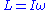) is the rotational analog of momentum
Momentum
In classical mechanics, linear momentum or translational momentum is the product of the mass and velocity of an object...

.
• Magnetic moment
Magnetic moment
The magnetic moment of a magnet is a quantity that determines the force that the magnet can exert on electric currents and the torque that a magnetic field will exert on it...

(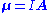) is a dipole
Dipole
In physics, there are several kinds of dipoles:*An electric dipole is a separation of positive and negative charges. The simplest example of this is a pair of electric charges of equal magnitude but opposite sign, separated by some distance. A permanent electric dipole is called an electret.*A...

moment measuring the strength and direction of a magnetic source.
• Electric dipole moment
Electric dipole moment
In physics, the electric dipole moment is a measure of the separation of positive and negative electrical charges in a system of charges, that is, a measure of the charge system's overall polarity with SI units of Coulomb-meter...

is a dipole moment measuring the charge difference and direction between two or more charges. For example, the electric dipole moment between a change of -q and q separated by a distance of d is (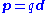)